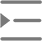# JS函数语法

• 2021-09-03 11:16:48
• 92次 动力节点

JavaScript函数是设计用于执行特定任务的代码块。

JavaScript函数在“某物”调用(调用它)时执行。

``````function myFunction(p1, p2) {
return p1 * p2;   // The function returns the product of p1 and p2
}``````

## JavaScript函数语法

JavaScript函数由function关键字定义，后跟名称，后跟括号()。

(参数1，参数2，...)

``````function name(parameter1, parameter2, parameter3) {
// code to be executed
}``````

## 函数返回

``````let x = myFunction(4, 3);   // Function is called, return value will end up in x
function myFunction(a, b) {
return a * b;             // Function returns the product of a and b
}``````

x中的结果将是：

``12``

## () 运算符调用函数

``````function toCelsius(fahrenheit) {
return (5/9) * (fahrenheit-32);
}
document.getElementById("demo").innerHTML = toCelsius;``````

## 用作变量值的函数

``````let x = toCelsius(77);
let text = "The temperature is " + x + " Celsius";``````

``let text = "The temperature is " + toCelsius(77) + " Celsius";``

## 局部变量

``````// code here can NOT use carName
function myFunction() {
let carName = "Volvo";
// code here CAN use carName
}
// code here can NOT use carName``````